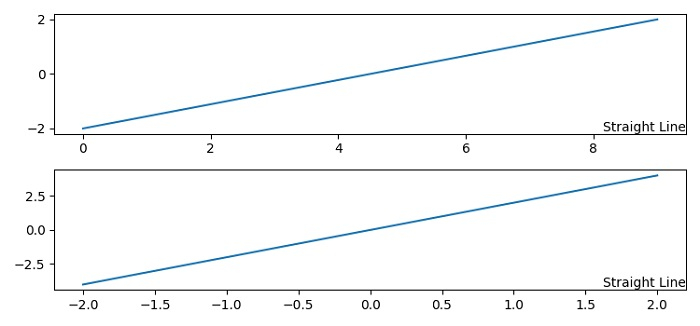# How to put text at the corner of an equal aspect figure in Python/Matplotlib?

To put text at the corner of an equal aspect figure in matplotlib, we can take the following steps −

• Set the figure size and adjust the padding between and around the subplots.

• Create a figure and a set of subplots, using subplots() method.

• Create x data points using numpy.

• Plot x on axis ax1, using plot() method.

• Plot x and 2*x on ax2, using plot() method.

• To put text in the corner of a figure use annotate() method for different axes.

• To display the figure, use show() method.

## Example

from matplotlib import pyplot as plt

plt.rcParams["figure.figsize"] = [7.50, 3.50]
plt.rcParams["figure.autolayout"] = True
fig, axes = plt.subplots(2)
x = np.linspace(-2, 2, 10)
axes.plot(x)
axes.plot(x, 2*x)
for ax in axes:
ax.annotate('Straight Line', xy=(1, 0),xycoords='axes fraction', fontsize=10, horizontalalignment='right', verticalalignment='bottom')
plt.show()

## Output